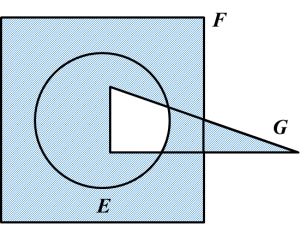# SPM Mathematics (Model Test Paper), Question 1 – 3

SPM Mathematics (Model Test Paper)

Section A
(52 marks)
Answer all questions in this section.

1. The Venn diagram in the answer space shows sets E, and G such that the universal set, $\xi =E\cup F\cup G.$
On the diagrams in the answer space, shade
$\begin{array}{l}\left(a\right)\text{}E\text{}\text{'}\cap G\text{'}\\ \left(b\right)\text{}\left(E\cap F\right)\text{'}\text{}\cup G\text{'}\end{array}$
[3 marks]
AnswerAnswer and solution:
(a)(b)2.   Calculate the value of x and of that satisfy the following simultaneous linear equations:
$\begin{array}{l}x+6y=12\\ \frac{2}{3}x+2y=6\end{array}$
[4 marks]
Answer and solution:
$\begin{array}{l}x+6y=12\\ x=12-6y---\left(1\right)\\ \frac{2}{3}x+2y=6\\ \left(×3\right)2x+6y=18\\ \left(÷2\right)x+3y=9---\left(2\right)\end{array}$

Substitute (1) into (2),
12 – 6y + 3= 9
–3y = –3
= 1
Substitute y = 1 into (1),
x = 12 – 6 (1)
x = 6

3.Diagram 1

Diagram 1 shows a square ABCD and a right-angled triangle PQR with equal area. Based on the information, find the value of x.
Hence, find the perimeter, in cm, of the square ABCD. [4 marks]

Answer and solution:

$\begin{array}{l}{\left(3x+2\right)}^{2}=\frac{1}{2}\left(4x+8\right)4x\\ 9{x}^{2}+12x+4=8{x}^{2}+16x\\ {x}^{2}-4x+4=0\\ \left(x-2\right)\left(x-2\right)=0\\ x=2\end{array}$

Perimeter of the square ABCD
= (3x + 2) × 4
= [3 (2) + 2] × 4
= 32 cm